#### 期刊菜单

The Numerical Analysis and Simulation Based on A Mixed Finite Element Method for the MRLW Equation
DOI: 10.12677/AAM.2019.812240, PDF, HTML, XML, 下载: 612  浏览: 750  科研立项经费支持

Abstract: A second-order backward-difference mixed finite element (MFE) method for modified regularized long wave (MRLW) equation is proposed and discussed in this paper. The spatial direction is approximated by the mixed Galerkin method using mixed linear space finite elements, and the time direction is considered by backward difference scheme with second-order convergence rate. The optimal error estimates for u in L2 and H1-norms and its flux q = ux and in L2-norm are derived. Some numerical results are given to test our theoretical analysis and illustrate the efficiency of the studied method.

1. 引言

$\left\{\begin{array}{l}{u}_{t}+{u}_{x}+6{u}^{2}{u}_{x}-\mu {u}_{xxt}=0,\text{ }\left(x,t\right)\in I×J\\ u\left(a,t\right)=u\left(b,t\right)=0,\text{ }t\in \stackrel{¯}{J},\\ u\left(x,0\right)={u}_{0}\left(x\right),\text{ }x\in \stackrel{¯}{I},\end{array}$ (1.1)

Mei和Chen  针对RLW方程研究了基于Galerkin方法的显式两步方法。在本文中，我们的目的是对修正RLW方程研究一种显式两步混合元法，利用混合Galerkin方法逼近空间方向，向后二阶差分格式逼近时间方向。我们在全离散显式两步混合格式下，得到了未知函数u在 ${L}^{2}$ 模和 ${H}^{1}$ 模下最优误差估计和其辅助变量 $q={u}_{x}$ 基于 ${L}^{2}$ 模最优误差估计，并与其他的数值方法的准确性进行了比较。通过与文献    中的数值方法进行比较，我们得到了u与 $q={u}_{x}$ 的近似值。

2. 混合有限元数值方法

${u}_{x}=q$ (2.1)

${u}_{t}+q+6{u}^{2}q-\mu {q}_{xt}=0.$ (2.2)

$\left({u}_{x},w\right)=\left(q,w\right),\forall w\in {L}^{2}\left(\Omega \right)$ (2.3)

$\left({u}_{t},v\right)+\left(q,v\right)+6\left({u}^{2}q,v\right)+\mu \left({q}_{t},{v}_{x}\right)=0,\forall v\in {H}_{0}^{1}$ .(2.4)

${V}_{h}=\left\{{v}_{h}|{v}_{h}\in {C}^{0}\left(\stackrel{¯}{I}\right),{{v}_{h}|}_{{I}_{j}}\in {P}_{k}\left({I}_{j}\right),\forall {I}_{j}\in {T}_{h},{v}_{h}\left(a\right)={v}_{h}\left(b\right)=0\right\}\subset {H}_{0}^{1},$

${W}_{h}=\left\{{w}_{h}|{w}_{h}\in {L}^{2}\left(\Omega \right),{{w}_{h}|}_{{I}_{j}}\in {P}_{r}\left({I}_{j}\right),\forall {I}_{j}\in {T}_{h}\right\}\subset {L}^{2}\left(\Omega \right),$

$\left({u}_{hx},{w}_{h}\right)=\left({q}_{h},{w}_{h}\right),\text{\hspace{0.17em}}\text{\hspace{0.17em}}\forall {w}_{h}\in {W}_{h},$ (2.5)

$\left({u}_{ht},{v}_{h}\right)+\left({q}_{h},{v}_{h}\right)+6\left({\left({u}_{h}\right)}^{2}{q}_{h},{v}_{h}\right)+\mu \left({q}_{ht},{v}_{hx}\right)=0,\text{\hspace{0.17em}}\text{\hspace{0.17em}}\forall {v}_{h}\in {V}_{h}.$ (2.6)

3. 二阶向后差分混合方法和最优误差估计

3.1. 二阶向后差分方法和相关定理

$\left({u}_{x}^{n+1},w\right)=\left({q}^{n+1},w\right),\text{ }\forall w\in {L}_{2}\left(\Omega \right),$ (3.1)

$\left({u}_{t}^{n+1},v\right)+\left({q}^{n+1},v\right)+6\left({\left({u}^{n+1}\right)}^{2}{q}^{n+1},v\right)+\mu \left({q}_{t}^{n+1},{v}_{x}\right)=0,\text{ }\forall v\in {H}_{0}^{1}.$ (3.2)

$\left({u}_{x}^{n+1},w\right)=\left({q}^{n+1},w\right),\text{ }\forall w\in {L}_{2}\left(I\right),$ (3.3)

$\begin{array}{l}\left({\partial }_{t}^{2}{u}^{n+1},v\right)+\mu \left({\partial }_{t}^{2}{q}^{n+1},{v}_{x}\right)+\left({q}^{n+1},v\right)+6\left(2{\left({u}^{n}\right)}^{2}{q}^{n}-{\left({u}^{n-1}\right)}^{2}{q}^{n-1},v\right)\\ =\left({\tau }_{1}^{n+1},v\right)+\left({\tau }_{2}^{n+1},{v}_{x}\right)+6\left({\tau }_{3}^{n+1},v\right),\text{ }\forall v\in {H}_{0}^{1},\end{array}$ (3.4)

$\begin{array}{l}{\tau }_{1}^{n+1}={\partial }_{t}^{2}{u}^{n+1}-{u}_{t}^{n+1},\\ {\tau }_{2}^{n+1}=\mu \left({\partial }_{t}^{2}{q}_{x}^{n+1}-{q}_{xt}^{n+1}\right),\\ {\tau }_{3}^{n+1}=\left(2{\left({u}^{n}\right)}^{2}{q}^{n}-{\left({u}^{n-1}\right)}^{2}{q}^{n-1}\right)-{\left({u}^{n+1}\right)}^{2}{q}^{n+1}.\end{array}$ (3.5)

$‖{\tau }_{1}^{n+1}‖+‖{\tau }_{2}^{n+1}‖+‖{\tau }_{3}^{n+1}‖\le C\Delta {t}^{2}.$ (3.6)

$\left({\partial }_{t}^{2}{w}^{n+1},{w}^{n+1}\right)\ge \frac{1}{4\Delta t}\left[{‖{w}^{n+1}‖}^{2}+{‖2{w}^{n+1}-{w}^{n}‖}^{2}-{‖{w}^{n}‖}^{2}-{‖2{w}^{n}-{w}^{n-1}‖}^{2}\right].$ (3.7)

$\left({u}_{hx}^{n+1},{w}_{h}\right)=\left({q}_{h}^{n+1},{w}_{h}\right),\text{ }\forall {w}_{h}\in {W}_{h},$ (3.8)

$\left({\partial }_{t}^{2}{u}_{h}^{n+1},{v}_{h}\right)+\mu \left({\partial }_{t}^{2}{q}_{h}^{n+1},{v}_{hx}\right)+\left({q}_{h}^{n+1},{v}_{h}\right)+6\left(2{\left({u}_{h}^{n}\right)}^{2}{q}_{h}^{n}-{\left({u}_{h}^{n-1}\right)}^{2}{q}_{h}^{n-1},{v}_{h}\right)=0,\text{ }\forall {v}_{h}\in {V}_{h}.$ (3.9)

$\left(q-{\Pi }_{h}q,{v}_{hx}\right)=0,\text{ }{v}_{h}\in {V}_{h},$ (3.10)

$‖q-{\Pi }_{h}q‖\le C{h}^{k+1}{‖q‖}_{k+1},$ (3.11)

$\left({u}_{x}-{P}_{h}{u}_{x},{w}_{h}\right)=0,\text{ }{w}_{h}\in {W}_{h},$ (3.12)

$‖{u}_{x}-{P}_{h}{u}_{x}‖+h{‖u-{P}_{h}u‖}_{1}\le C{h}^{k+1}{‖u‖}_{k+1}$ (3.13)

${u}^{n}-{u}_{h}^{n}=\left({u}^{n}-{P}_{h}{u}^{n}\right)+\left({P}_{h}{u}^{n}-{u}_{h}^{n}\right)={\eta }^{n}+{\varsigma }^{n},$

${q}^{n}-{q}_{h}^{n}=\left({q}^{n}-{\Pi }_{h}{q}^{n}\right)+\left({\Pi }_{h}{q}^{n}-{q}_{h}^{n}\right)={\rho }^{n}+{\xi }^{n}.$

$\left({\xi }^{n+1}+{\rho }^{n+1},{w}_{h}\right)=\left({\varsigma }_{x}^{n+1},{w}_{h}\right),\text{ }\forall {w}_{h}\in {W}_{h},$ (3.14)

$\begin{array}{l}\left({\partial }_{t}^{2}{\varsigma }^{n+1},{v}_{h}\right)+\mu \left({\partial }_{t}^{2}{\xi }^{n+1},{v}_{hx}\right)+6\left(2\left({\left({u}^{n}\right)}^{2}{q}^{n}-{\left({u}_{h}^{n}\right)}^{2}{q}_{h}^{n}\right)-\left({\left({u}^{n-1}\right)}^{2}{q}^{n-1}-{\left({u}_{h}^{n-1}\right)}^{2}{q}_{h}^{n-1}\right),{v}_{h}\right)\\ +\left({\rho }^{n+1}+{\xi }^{n+1},{v}_{h}\right)=-\left({\partial }_{t}^{2}{\eta }^{n+1},{v}_{h}\right)+\left({\tau }_{1}^{n+1},{v}_{h}\right)+\left({\tau }_{2}^{n+1},{v}_{hx}\right)+6\left({\tau }_{3}^{n+1},{v}_{h}\right),\text{ }{v}_{h}\in {V}_{h}.\end{array}$ (3.15)

3.2. 最优误差估计

$\begin{array}{l}‖{u}^{n}-{u}_{h}^{n}‖+‖{q}^{n}-{q}_{h}^{n}‖\le C\left({h}^{k+1}+\Delta {t}^{2}\right),\\ {‖{u}^{n}-{u}_{h}^{n}‖}_{1}\le C\left({h}^{k}+\Delta {t}^{2}\right).\end{array}$

$\left({\partial }_{t}^{2}{\xi }^{n+1},{w}_{h}\right)=\left({\partial }_{t}^{2}{\varsigma }_{x}^{n+1},{w}_{h}\right)-\left({\partial }_{t}^{2}{\rho }^{n+1},{w}_{h}\right),\text{ }\forall {w}_{h}\in {W}_{h},$ (3.16)

$\begin{array}{l}\left({\partial }_{t}^{2}{\varsigma }^{n+1},{\varsigma }^{n+1}\right)+\mu \left({\partial }_{t}^{2}{\varsigma }_{x}^{n+1},{\varsigma }_{x}^{n+1}\right)\\ =\mu \left({\partial }_{t}^{2}{\rho }^{n+1},{\varsigma }_{x}^{n+1}\right)-6\left(2\left({\left({u}^{n}\right)}^{2}{q}^{n}-{\left({u}_{h}^{n}\right)}^{2}{q}_{h}^{n}\right)-\left({\left({u}^{n-1}\right)}^{2}{q}^{n-1}-{\left({u}_{h}^{n-1}\right)}^{2}{q}_{h}^{n-1}\right),{\varsigma }^{n+1}\right)\\ \text{ }+\left({\rho }^{n+1}+{\xi }^{n+1},{\varsigma }^{n+1}\right)+\left({\tau }_{1}^{n+1},{\varsigma }^{n+1}\right)+\left({\tau }_{2}^{n+1},{\varsigma }_{x}^{n+1}\right)+6\left({\tau }_{3}^{n+1},{\varsigma }^{n+1}\right)\\ \le C\left({‖2\left({\left({u}^{n}\right)}^{2}{q}^{n}-{\left({u}_{h}^{n}\right)}^{2}{q}_{h}^{n}\right)-\left({\left({u}^{n-1}\right)}^{2}{q}^{n-1}-{\left({u}_{h}^{n-1}\right)}^{2}{q}_{h}^{n-1}\right)‖}^{2}\\ \text{\hspace{0.17em}}\text{\hspace{0.17em}}\begin{array}{c}\text{ }\\ \text{ }\end{array}+{‖{\rho }^{n+1}‖}^{2}+{‖{\xi }^{n+1}‖}^{2}+{‖{\tau }_{1}^{n+1}‖}^{2}+{‖{\tau }_{2}^{n+1}‖}^{2}+{‖{\tau }_{3}^{n+1}‖}^{2}+{‖{\varsigma }^{n+1}‖}^{2}+{‖{\varsigma }_{x}^{n+1}‖}^{2}\right).\end{array}$ (3.17)

$\begin{array}{l}{‖2\left({\left({u}^{n}\right)}^{2}{q}^{n}-{\left({u}_{h}^{n}\right)}^{2}{q}_{h}^{n}\right)-\left({\left({u}^{n-1}\right)}^{2}{q}^{n-1}-{\left({u}_{h}^{n-1}\right)}^{2}{q}_{h}^{n-1}\right)‖}^{2}\\ \le {‖2{\left({u}^{n}\right)}^{2}\left({q}^{n}-{q}_{h}^{n}\right)+2\left({\left({u}^{n}\right)}^{2}-{\left({u}_{h}^{n}\right)}^{2}\right){q}_{h}^{n}-\left({\left({u}^{n-1}\right)}^{2}\left({q}^{n-1}-{q}_{h}^{n-1}\right)+\left[{\left({u}^{n-1}\right)}^{2}-{\left({u}_{h}^{n-1}\right)}^{2}\right]{q}_{h}^{n-1}\right)‖}^{2}\\ \le C\underset{j=n-1}{\overset{n}{\sum }}\left[{‖{\left({u}^{j}\right)}^{2}‖}_{\infty }^{2}{\left(‖{\xi }^{j}‖+‖{\rho }^{j}‖\right)}^{2}+{\left({‖{u}^{j}‖}_{\infty }+{‖{u}_{h}^{j}‖}_{\infty }\right)}^{2}{‖{q}_{h}^{j}‖}_{\infty }^{2}{\left(‖{\eta }^{j}‖+‖{\varsigma }^{j}‖\right)}^{2}\right]\\ \le C\left({‖{\rho }^{n}‖}^{2}+{‖{\rho }^{n-1}‖}^{2}+{‖{\eta }^{n}‖}^{2}+{‖{\eta }^{n-1}‖}^{2}+{‖{\xi }^{n}‖}^{2}+{‖{\xi }^{n-1}‖}^{2}+{‖{\varsigma }^{n}‖}^{2}+{‖{\varsigma }^{n-1}‖}^{2}\right).\end{array}$ (3.18)

${‖{\xi }^{n+1}‖}^{2}\le C\left({‖{\rho }^{n+1}‖}^{2}+{‖{\varsigma }_{x}^{n+1}‖}^{2}\right).$ (3.19)

$\begin{array}{l}\left({\partial }_{t}^{2}{\varsigma }^{n+1},{\varsigma }^{n+1}\right)+\mu \left({\partial }_{t}^{2}{\varsigma }_{x}^{n+1},{\varsigma }_{x}^{n+1}\right)\\ =\mu \left({\partial }_{t}^{2}{\rho }^{n+1},{\varsigma }_{x}^{n+1}\right)-6\left(2\left({\left({u}^{n}\right)}^{2}{q}^{n}-{\left({u}_{h}^{n}\right)}^{2}{q}_{h}^{n}\right)-\left({\left({u}^{n-1}\right)}^{2}{q}^{n-1}-{\left({u}_{h}^{n-1}\right)}^{2}{q}_{h}^{n-1}\right),{\varsigma }^{n+1}\right)\\ \text{ }+\left({\rho }^{n+1}+{\xi }^{n+1},{\varsigma }^{n+1}\right)+\left({\tau }_{1}^{n+1},{\varsigma }^{n+1}\right)+\left({\tau }_{2}^{n+1},{\varsigma }_{x}^{n+1}\right)+6\left({\tau }_{3}^{n+1},{\varsigma }^{n+1}\right)\\ \le C\left({‖{\rho }^{n+1}‖}^{2}+{‖{\tau }_{1}^{n+1}‖}^{2}+{‖{\tau }_{2}^{n+1}‖}^{2}+{‖{\tau }_{3}^{n+1}‖}^{2}+\underset{i=n-1}{\overset{n+1}{\sum }}\left({‖{\varsigma }^{i}‖}^{2}+{‖{\varsigma }_{x}^{i}‖}^{2}\right)\\ \text{\hspace{0.17em}}\text{\hspace{0.17em}}\begin{array}{c}\text{ }\\ \text{ }\end{array}+{‖{\partial }_{t}^{2}{\rho }^{n+1}‖}^{2}+{‖{\rho }^{n}‖}^{2}+{‖{\rho }^{n-1}‖}^{2}+{‖{\eta }^{n}‖}^{2}+{‖{\eta }^{n-1}‖}^{2}\right).\end{array}$ (3.20)

$\begin{array}{l}{‖{\varsigma }^{n+1}‖}_{1}^{2}+{‖2{\varsigma }^{n+1}-{\varsigma }^{n}‖}_{1}^{2}-{‖{\varsigma }^{n}‖}_{1}^{2}-{‖2{\varsigma }^{n}-{\varsigma }^{n-1}‖}_{1}^{2}\\ \le C\left({‖{\rho }^{n+1}‖}^{2}+{‖{\tau }_{1}^{n+1}‖}^{2}+{‖{\tau }_{2}^{n+1}‖}^{2}+{‖{\tau }_{3}^{n+1}‖}^{2}+\underset{i=n-1}{\overset{n+1}{\sum }}\left({‖{\varsigma }^{i}‖}^{2}+{‖{\varsigma }_{x}^{i}‖}^{2}\right)\\ \begin{array}{c}\\ \end{array}+{‖{\partial }_{t}^{2}{\rho }^{n+1}‖}^{2}+{‖{\rho }^{n}‖}^{2}+{‖{\rho }^{n-1}‖}^{2}+{‖{\eta }^{n}‖}^{2}+{‖{\eta }^{n-1}‖}^{2}\right).\end{array}$ (3.21)

$\begin{array}{l}{‖{\varsigma }^{n+1}‖}_{1}^{2}+{‖2{\varsigma }^{n+1}-{\varsigma }^{n}‖}_{1}^{2}-{‖{\varsigma }^{n}‖}_{1}^{2}-{‖2{\varsigma }^{n}-{\varsigma }^{n-1}‖}_{1}^{2}\\ \le C\left({‖{\rho }^{n+1}‖}^{2}+{‖{\tau }_{1}^{n+1}‖}^{2}+{‖{\tau }_{2}^{n+1}‖}^{2}+{‖{\tau }_{3}^{n+1}‖}^{2}\\ \text{\hspace{0.17em}}\text{\hspace{0.17em}}+{‖{\partial }_{t}^{2}{\rho }^{n+1}‖}^{2}+{‖{\rho }^{n}‖}^{2}+{‖{\rho }^{n-1}‖}^{2}+{‖{\eta }^{n}‖}^{2}+{‖{\eta }^{n-1}‖}^{2}\right).\end{array}$ (3.22)

$\begin{array}{c}{‖{\xi }^{n+1}‖}^{2}\le C\left({‖{\rho }^{n+1}‖}^{2}+{‖{\tau }_{1}^{n+1}‖}^{2}+{‖{\tau }_{2}^{n+1}‖}^{2}+{‖{\tau }_{3}^{n+1}‖}^{2}\\ \text{\hspace{0.17em}}\text{\hspace{0.17em}}+{‖{\partial }_{t}^{2}{\rho }^{n+1}‖}^{2}+{‖{\rho }^{n}‖}^{2}+{‖{\rho }^{n-1}‖}^{2}+{‖{\eta }^{n}‖}^{2}+{‖{\eta }^{n-1}‖}^{2}\right).\end{array}$ (3.23)

4. 数值实验

$\begin{array}{l}{I}_{1}={\int }_{a}^{b}u\text{d}x\approx h\underset{j=1}{\overset{N}{\sum }}{u}_{j}^{n},\\ {I}_{2}={\int }_{a}^{b}\left({u}^{2}+\mu {\left({u}_{x}\right)}^{2}\right)\text{d}x\approx h\underset{j=1}{\overset{N}{\sum }}\left[{\left({u}_{j}^{n}\right)}^{2}+\mu {\left[{\left({u}_{x}\right)}_{j}^{n}\right]}^{2}\right]\text{\hspace{0.17em}},\\ {I}_{3}={\int }_{a}^{b}\left({u}^{4}-\mu {\left({u}_{x}\right)}^{2}\right)\text{d}x\approx h\underset{j=1}{\overset{N}{\sum }}\left[{\left({u}_{j}^{n}\right)}^{4}-\mu {\left[{\left({u}_{x}\right)}_{j}^{n}\right]}^{2}\right]\text{\hspace{0.17em}}.\end{array}$

$u\left(x,t\right)=\sqrt{c}\text{ }\text{sech}\left(p\left(x-\left(c+1\right)t-{x}_{0}\right)\right)$ (4.1)

${I}_{1}=\frac{\pi \sqrt{c}}{p},{I}_{2}=\frac{2c}{p}+\frac{2\mu pc}{3},{I}_{3}=\frac{4{c}^{2}}{3p}-\frac{2\mu pc}{3}.$

$u\left(x,0\right)=\sqrt{c}\text{ }\text{sech}\left(p\left(x-{x}_{0}\right)\right)$ (4.2)Table 1. Invariants of single solitary wave. h = 0 , 125 , Δ t = 0.0125 , c 1 = 1 , c 2 = 0.5Table 2. Convergence order and error in L2-norm for u of time. h = 0.125 , c = 1Table 3. Convergence order and error in L2-norm for q of time. h = 0. 125 , c = 1Table 4. Convergence order and error in L2-norm for u of space. Δ t = 0.01 , c = 1Table 5. Convergence order and error in L2-norm for q of space. Δ t = 0.01 , c = 1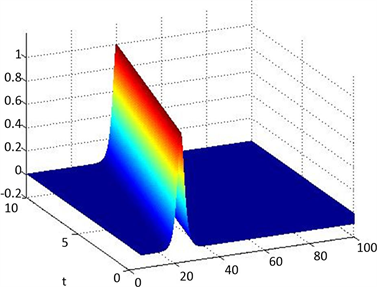Figure 1. Surface for exact solution u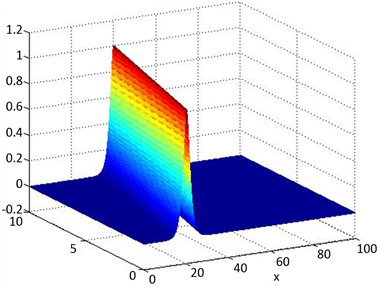Figure 2. Surface for numerical solution uhFigure 3. Surface for exact solution q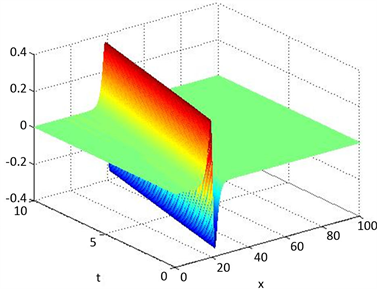Figure 4. Surface for numerical solution qh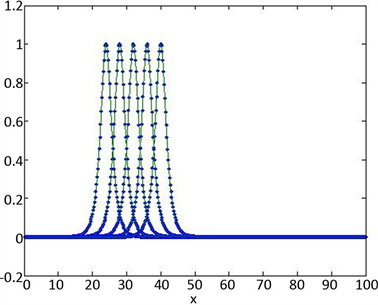Figure 5. Comparison between u and uh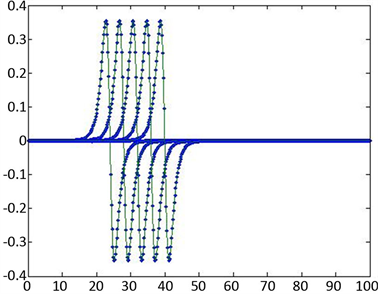Figure 6. Comparison between q and qh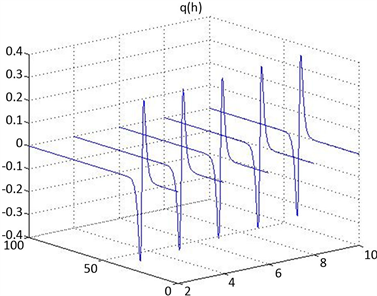Figure 7. Numerical solution uh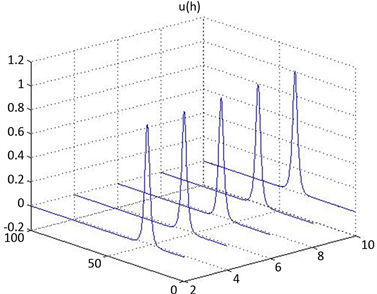Figure 8. Numerical solution qh

5. 结论

  Shi, D.Y. and Yang, H. (2016) A New Approach of Superconvergence Analysis for Nonlinear BBM Equation on Anisotropic Meshes. Applied Mathematics Letters, 58, 74-80. https://doi.org/10.1016/j.aml.2016.02.007  Benjamin, T.B., Bona, J.L. and Mahony, J.J. (1972) Model Equations for Long Waves in Nonlinear Dispersive Systems. Philosophical Transactions of the Royal Society of London. Series A, Mathematical and Physical Sciences, 272, 47-78. https://doi.org/10.1098/rsta.1972.0032  Olver, P.J. (1979) Euler Operators and Conservation Laws of the BBM Equation. Mathematical Proceedings of the Cambridge Philosophical Society, 85, 143-160. https://doi.org/10.1017/S0305004100055572  Saka, B., Şahin, A. and Dağ, İ. (2011) B-Spline Collocation Algorithms for Numerical Solution of the RLW Equation. Numerical Methods for Partial Differential Equations, 27, 581-607. https://doi.org/10.1002/num.20540  Bona, J.L. and Bryant, P.J. (1973) A Mathematical Model for Long Waves Generated by Wavemakers in Non-Linear Dispersive Systems. Mathematical Proceedings of the Cambridge Philosophical Society, 73, 391-405. https://doi.org/10.1017/S0305004100076945  Zhang, L. (2005) A Finite Difference Scheme for Generalized Regularized Long-Wave Equation. Applied Mathematics and Computation, 168, 962-972. https://doi.org/10.1016/j.amc.2004.09.027  Dagˇ, İ., DoĞan, A. and Saka, B. (2003) B-Spline Collocation Methods for Numerical Solutions of the RLW Equation. International Journal of Computer Mathematics, 80, 743-757. https://doi.org/10.1080/0020716021000038965  Esen, A. and Kutluay, S. (2006) Application of a Lumped Galerkin Method to the Regularized Long Wave Equation. Applied Mathematics and Computation, 174, 833-845. https://doi.org/10.1016/j.amc.2005.05.032  Dağ, İ., Saka, B. and Irk, D. (2006) Galerkin Method for the Numerical Solution of the RLW Equation Using Quintic B-Splines. Journal of Computational and Applied Mathematics, 190, 532-547. https://doi.org/10.1016/j.cam.2005.04.026  Raslan, K.R. (2005) A Computational Method for the Regularized Long Wave (RLW) Equation. Applied Mathematics and Computation, 167, 1101-1118. https://doi.org/10.1016/j.amc.2004.06.130  Wahlbin, L. (1974) Error Estimates for a Galerkin Method for a Class of Model Equations for Long Waves. Numerische Mathematik, 23, 289-303. https://doi.org/10.1007/BF01438256  Luo, Z. and Liu, R. (1998) Mixed Finite Element Analysis and Numerical Solitary Solution for the RLW Equation. SIAM Journal on Numerical Analysis, 36, 89-104. https://doi.org/10.1137/S0036142996312999  Guo, L. and Chen, H. (2006) H1-Galerkin Mixed Finite Element Method for the Regularized Long Wave Equation. Computing, 77, 205-221. https://doi.org/10.1007/s00607-005-0158-7  Gu, H. and Chen, N. (2008) Least-Squares Mixed Finite Element Methods for the RLW Equations. Numerical Methods for Partial Differential Equations: An International Journal, 24, 749-758. https://doi.org/10.1002/num.20285  Bona, J.L., Pritchard, W.G. and Scott, L.R. (1985) Numerical Schemes for a Model for Nonlinear Dispersive Waves. Journal of Computational Physics, 60, 167-186. https://doi.org/10.1016/0021-9991(85)90001-4  Haq, S. and Ali, A. (2009) A Meshfree Method for the Numerical Solution of the RLW Equation. Journal of Computational and Applied Mathematics, 223, 997-1012. https://doi.org/10.1016/j.cam.2008.03.039  陈邵春, 陈红如. 二阶椭圆问题新的混合元格式[J]. 计算数学, 2010, 32(2): 213-218.  Mei, L. and Chen, Y. (2012) Explicit Multistep Method for the Numerical Solution of RLW Equation. Applied Mathematics and Computation, 218, 9547-9554. https://doi.org/10.1016/j.amc.2012.03.050  Gardner, L.R.T., Gardner, G.A. and Dogan, A. (1996) A Least-Squares Finite Element Scheme for the RLW Equation. Communications in Numerical Methods in Engineering, 12, 795-804. https://doi.org/10.1002/(SICI)1099-0887(199611)12:11<795::AID-CNM22>3.0.CO;2-O  Daǧ, İ. (2000) Least-Squares Quadratic B-Spline Finite Element Method for the Regularised Long Wave Equation. Computer Methods in Applied Mechanics and Engineering, 182, 205-215. https://doi.org/10.1016/S0045-7825(99)00106-1  Daǧ, İ. and Özer, M.N. (2001) Approximation of the RLW Equation by the Least Square Cubic B-Spline Finite Element Method. Applied Mathematical Modelling, 25, 221-231. https://doi.org/10.1016/S0307-904X(00)00030-5  Dogan, A. (2002) Numerical Solution of RLW Equation Using Linear Finite Elements within Galerkin’s Method. Applied Mathematical Modelling, 26, 771-783. https://doi.org/10.1016/S0307-904X(01)00084-1  刘洋, 李宏. 偏微分方程的非标准混合有限元方法[M]. 北京: 国防工业出版社, 2015.  Chatzipantelidis, P. (1998) Explicit Multistep Methods for Nonstiff Partial Differential Equations. Applied Numerical Mathematics, 27, 13-31. https://doi.org/10.1016/S0168-9274(97)00112-8  Akrivis, G., Karakashian, O. and Karakatsani, F. (2003) Linearly Implicit Methods for Nonlinear Evolution Equations. Numerische Mathematik, 94, 403-418. https://doi.org/10.1007/s00211-002-0432-y  Liu, Y., Li, H., Du, Y. and Wang, J. (2013) Explicit Multistep Mixed Finite Element Method for RLW Equation. Abstract and Applied Analysis, 2013, Article ID: 768976. https://doi.org/10.1155/2013/768976  Liu, Y., Li, H., Gao, W., He, S. and Fang, Z. (2014) A New Mixed Element Method for a Class of Time-Fractional Partial Differential Equations. The Scientific World Journal, 2014, Article ID: 141467. https://doi.org/10.1155/2014/141467